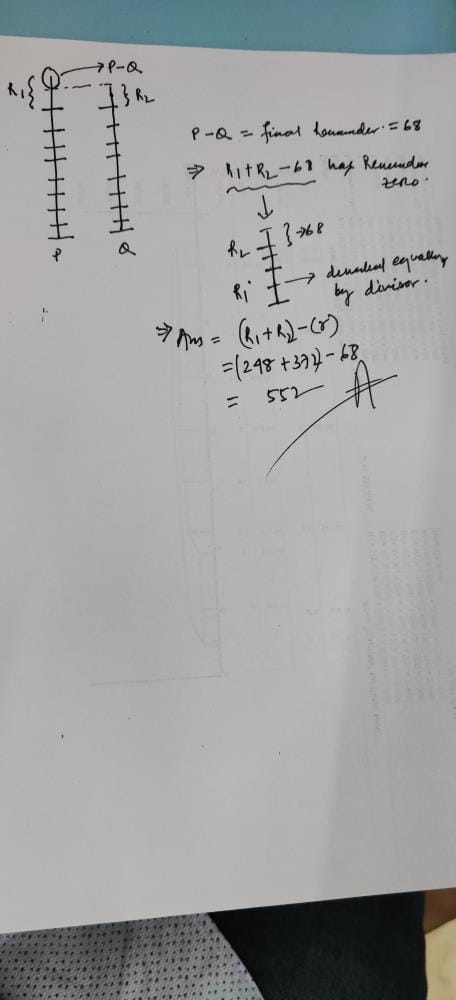Two numbers when divided by a divisor leave a remainder of 248 and 372 respectively. The remainder obtained when the sum of the numbers is divided by same divisor is 68. What is the divisor?
• a)
276
• b)
552
• c)
414
• d)
1004

### Related TestPreitee Ranjan Pradhan Jun 01, 2020